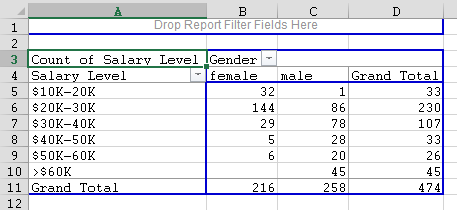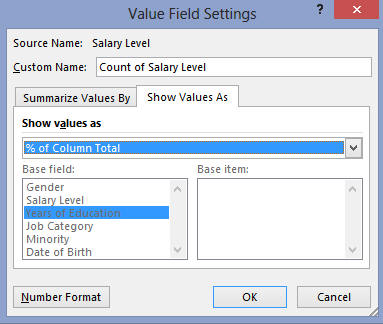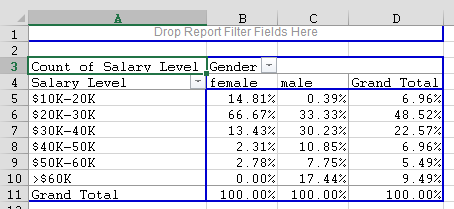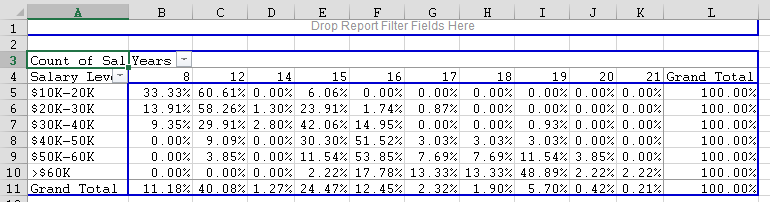#MathCS.org - Statistics

## 5.3 Crosstabs Tables

In the first part of this chapter we saw how to use Excel's "Pivot" function to compute counts or frequencies for us, using one (categorical) variable at a time. In the second part we saw how to create row and column percentage tables for two categorical variables manually, if we already knew the total counts in the respective categories. Now we want to automatically generate tables involving two variables from a particular data set instead of converting existing tables into percentage tables. The procedure will be similar to the one before, so make sure you are familiar with the previous section.

Example: Attached is an Excel data file listing salary levels and other information about employees of a particular company. Create and interpret tables relating:

1. Gender with Salary Level
2. Salary Level with Years of Education
3. Salary Level with Job Category

With Excel, the appropriate tool to create such crosstabs tables is - you guessed it - the "PivotTable ..." in the "Insert" ribbon.

• Click "Insert", then "PivotTable ...", as in the previous section.
• Use the range selector tool to select the entire data set

You will see the table (hopefully) familiar from the previous section, where you can drag variables from the PivotTable Fields into the "Row", "Column", and/or "Values" areas. We won't make use of "Filters".

To analyze the relationship - if any - between "Gender" and "Salary Level", drag the variable "Salary Level" to the "Row" field of the table and the variable "Gender" to the "Column" field (if you accidentally drop a variable in the wrong spot, simply drag it back).That will create a table with "Salary levels" as rows and "Gender" as columns, but containing no data yet. Finally, drag either "Gender" or "Salary Level" into the "Data" field in the middle (in our case it does not matter which one but in the picture below we used "Salary Level") and the table will be complete - but with raw data, not percentages.This table shows, for example, that 32 female employees out of 474 total employees earn between \$10,000 and \$20,000, while, for example, 45 male employees earn more than \$60,000.

Similarly, we can create tables to relate "Salary Level" with "Years of Education". We could start again from scratch, but since we already have the pivot table available, we can simply drag the "Salary Level" and "Gender" variables out of the table and drag the "Years of Education" and "Salary Level" to the respective row, column, and data area to create our next table:Similarly, we can create the table relating "Salary Level" with "Job Category", but I leave the details up to you. To finish our discussion on crosstabs tables, let's use the above tables to answer specific questions:

Example: Using the same Excel data as before, answer the following questions:

1. How many female employees earn less than \$40,000 ? How many males ?
2. How many people earning more that \$60,000 have 15 years of eduation or less ? How many of those have more than 15 years of education ?

All answers should be in percent.

At first glance it might seem that we have already created the right tables to answer these questions. However, this time we want the answers in percent, while our above tables contain actual numbers. And, as we have discussed in the previous section, when we want to generate percentage tables we need to decide whether we want row or column percentages. Therefore, we first need to discuss exactly which tables to generate to answer these questions before worrying about how to do it in Excel.

To answer question 1, we clearly need to generate a table relating salary with gender. Let's use salary as row variable and gender as column, just as above:Question 1 uses as total all female employees, and in our table the "females" go along a column. Therefore, we need to generate column percentages in the above table.

• Double-click the "Count of Salary Level" button in your table and click on "Options" in the dialog that pops up. Then select "% of column" in the "Show Values As" field and click OK.You should see the final table, containing column percentages, as shown below. Note that you can see that the table shows column percentages since the percentages accross the columns add up to 100%.Now we can answer question 1 easily: Recall the question was "how many female employees earn less than \$40,000". In the female column we need to add the numbers: 14.81% + 66.67%+13.43% since everyone in those corresponding cells is female and earns less than \$40,000. You could of course use Excel to add these numbers, or simply add them in your head. The answer to the question is: 94.91% of females earn less than \$40,000. For the male employees, the answer is: 63.95% of males earn less than \$40,000.

Actually, this seems to indicate that female employees, as a rule, earn less money than male employees. We will learn how to answer questions such as these in the next section.

It is left as an exercise to answer the second set of questions "How many people earning more than \$60,000 have 15 years of education or less ? How many of those have more than 15 years of education". Here are, for your information, the answers (recall that you first need to determine whether to use row or column percentage tables, which of course depends on your choice of row and column variables, as well as on the particular question. Here is a particular table we chose to create:By rthe ways, is that table using row or column percentages ? The correct answers are:

• 2.22% of people earning more than \$60,000 have 15 years or less of education
• 97.78% of people earning more than \$60,000 have more than 15 years of education

Again, a more interesting question would be be to determine whether more years of education generally result in higher salaries (it does looks that way) - we will answer that type of question in the next section.